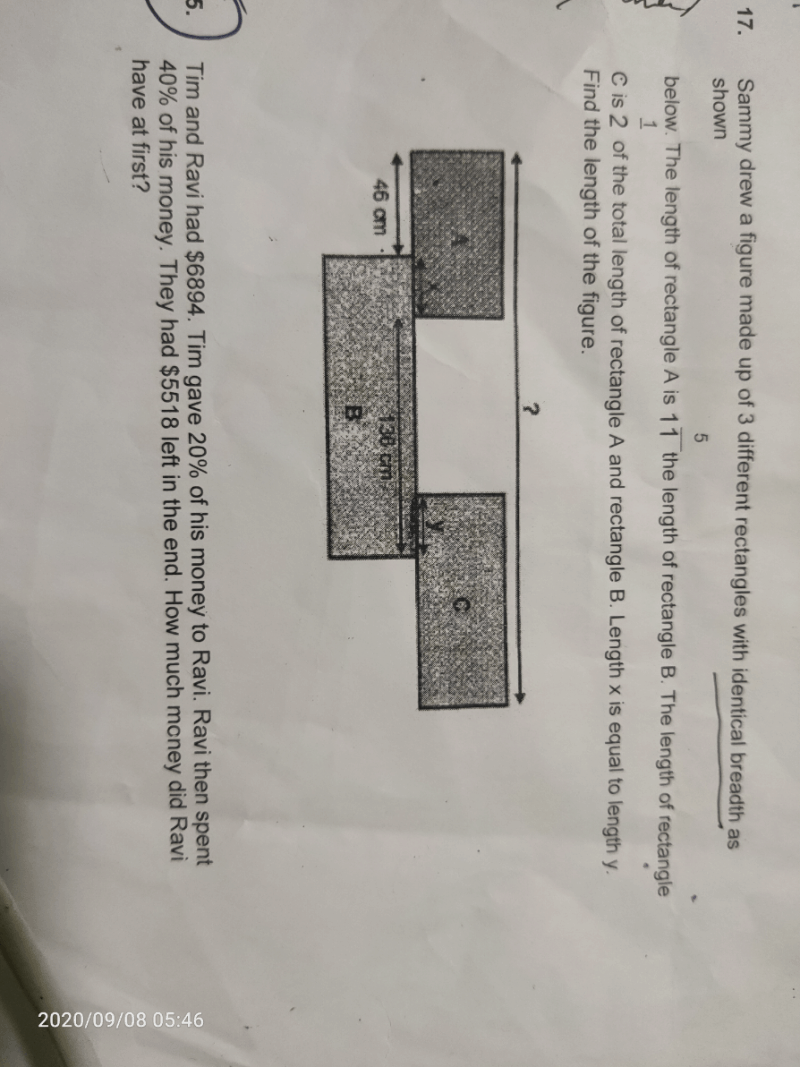# QuestionLength of A → 5u

Length of B → 11u

Length of C → ½ × (5u + 11u) = 8u

5u = 46 + x

x = 5u – 46

11u = 136 + x

x = 11u – 136

5u – 46 = 11u – 136

6u = 90

1u = 15

8u = 15 × 8 = 120

Length of figure → 46 + 136 + 120 = 302 cm